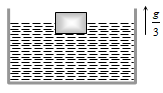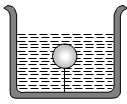A concrete sphere of radius R has a cavity of radius r which is packed with sawdust. The specific gravities of concrete and sawdust are respectively 2.4 and 0.3 for this sphere to float with its entire volume submerged under water. Ratio of mass of concrete to mass of sawdust will be
(a) 8                                        (b) 4
(c) 3                                        (d) Zero

Concept Questions :-

Properties of fluids
High Yielding Test Series + Question Bank - NEET 2020

Difficulty Level:

A metallic block of density 5 gm ${\mathrm{cm}}^{-3}$ and having dimensions 5 cm × 5 cm × 5 cm is weighed in water. Its apparent weight will be
(a) 5 × 5 × 5 × 5 gf                            (b) 4 × 4 × 4 × 4 gf
(c) 5 × 4 × 4 × 4 gf                            (d) 4 × 5 × 5 × 5 gf

Concept Questions :-

Archimedes principle
High Yielding Test Series + Question Bank - NEET 2020

Difficulty Level:

A cubical block is floating in a liquid with half of its volume immersed in the liquid. When the whole system accelerates upwards with acceleration of g/3, the fraction of volume immersed in the liquid will be(a) $\frac{1}{2}$                                  (b) $\frac{3}{8}$

(c) $\frac{2}{3}$                                  (d) $\frac{3}{4}$

Concept Questions :-

Archimedes principle
High Yielding Test Series + Question Bank - NEET 2020

Difficulty Level:

A silver ingot weighing 2.1 kg is held by a string so as to be completely immersed in a liquid of relative density 0.8. The relative density of silver is 10.5. The tension in the string in kg-wt is
(a) 1.6                                             (b) 1.94
(c) 3.1                                             (d) 5.25

Concept Questions :-

Properties of fluids
High Yielding Test Series + Question Bank - NEET 2020

Difficulty Level:

Two solids A and B float in water. It is observed that A floats with half its volume immersed and B floats with 2/3 of its volume immersed. Compare the densities of A and B

(a) 4:3                         (b) 2:3

(c) 3:4                         (d) 1:3

Concept Questions :-

Archimedes principle
High Yielding Test Series + Question Bank - NEET 2020

Difficulty Level:

The fraction of a floating object of volume ${\mathrm{V}}_{0}$ and density ${\mathrm{d}}_{0}$ above the surface of a liquid of density d will be

(a) $\frac{{\mathrm{d}}_{0}}{\mathrm{d}}$                                       (b) $\frac{{\mathrm{dd}}_{0}}{\mathrm{d}+{\mathrm{d}}_{0}}$

(c) $\frac{\mathrm{d}-{\mathrm{d}}_{0}}{\mathrm{d}}$                                   (d) $\frac{{\mathrm{dd}}_{0}}{\mathrm{d}-{\mathrm{d}}_{0}}$

Concept Questions :-

Archimedes principle
High Yielding Test Series + Question Bank - NEET 2020

Difficulty Level:

Pressure applied to an enclosed fluid is transmitted undiminished to every portion of the fluid and the walls of the containing vessel. This law was first formulated by:

(a) Bernoulli                                (b) Archimedes
(c) Boyle                                     (d) Pascal

Concept Questions :-

Pressure
High Yielding Test Series + Question Bank - NEET 2020

Difficulty Level:

A body is just floating on the surface of a liquid. The density of the body is same as that of the liquid. The body is slightly pushed down. What will happen to the body ?
(a) It will slowly come back to its earlier position

(b) It will remain submerged, where it is left

(c) It will sink

(d) It will come out violently

Concept Questions :-

Archimedes principle
High Yielding Test Series + Question Bank - NEET 2020

Difficulty Level:

A cork is submerged in water by a spring attached to the bottom of a bowl. When the bowl is kept in an elevator moving with acceleration downwards, the length of spring

(a) Increases                                          (b) Decreases
(c) Remains unchanged                           (d) None of these

Concept Questions :-

Archimedes principle
High Yielding Test Series + Question Bank - NEET 2020

Difficulty Level:

A solid sphere of density $\mathrm{\eta }$ ( > 1) times lighter than water is suspended in a water tank by a string tied to its base as shown in fig. If the mass of the sphere is m then the tension in the string is given by(a) $\left(\frac{\mathrm{\eta }-1}{\mathrm{\eta }}\right)\mathrm{mg}$                              (b) $\mathrm{\eta mg}$

(c) $\frac{mg}{\mathrm{\eta }}$                                        (d) $\left(\mathrm{\eta }-1\right)\mathrm{mg}$

Concept Questions :-

Archimedes principle